# AP Chemistry Practice Test 24

### Test Information10 questions15 minutes

1. What is the pKa of the methylammonium ion, CH3NH3+, which is the conjugate acid of methylamine in the previous question?

`Questions 2-5 refer to the following information.VesselABCGasBromomethaneBromoethane1-BromopropaneFormulaCH3BrCH3CH2BrCH3CH2CH2 BrMolar mass95 g/mol109 g/mol123 g/molPressure0.4 atm0.3 atm0.2 atmTemperature398 K398 K398 KNote that in addition to the data in the table, the gases are held in separate, identical, rigid vessels.`

2. The sample with the lowest density is

3. The average kinetic energy

4. Which of these gases will condense at the lowest pressure, assuming that the temperature is held constant?

5. Which attractive force is least likely to be the cause of condensation?

`Questions 6-7 refer to the following information.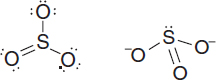`

6. The Lewis electron-dot structures for sulfur trioxide and for the sulfite ion are given above. Which statement best describes the geometry of these two substances?

7. Which statement below describes the charge, polarity, and resonance characteristics of the sulfite ion and of the sulfur trioxide species shown above?

`Questions 8-10 refer to the following information.Pb2+(aq) + 2Cl-(aq) → PbCl2(s)A chemist mixes a dilute solution of lead(II) perchlorate with an excess of potassium chloride to precipitate lead(II) chloride (Ksp = 1.7 × 10-5). The net ionic equation is given above for the reaction.`

8. Which of the following is a molecular equation for this reaction?

9. Which of the following particulate views represents the experiment after the reactants are mixed thoroughly and the solids are given time to precipitate?

 A.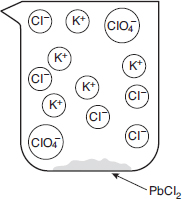B.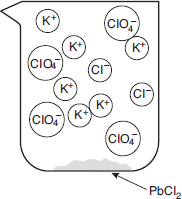C.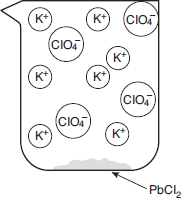D.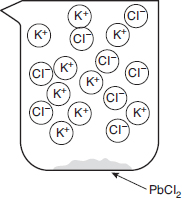10. Within a factor of ten, what is the approximate molar solubility of lead(II) chloride in distilled water? A. 1.7 × 10-5 mol/L B. 10-7 mol/L C. 10-2 mol/L D. 10-15 mol/L *AP & Advanced Placement Program are registered trademarks of the College Board, which was not involved in the production of, and does not endorse this site. All content of site and practice tests copyright © 2017 Max. sitemap Home Privacy Copyright Contact Link to us